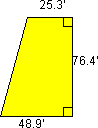NAME_________________________________ CLASS____________________ DATE___________________

Beginner's Guide to Aerodynamics

Drag Equation - Level 1

Worksheet

1. Click on The Drag Equation to open the appropriate slide. Study the equation and read the explanation of this concept. Then use the information to complete the questions below.

2. In the following table, identify each variable in the equation. State the appropriate units for each value:

#### Metric Units

D

Cd

r

V

A

A. What possible reference areas (A) can be used to compute the drag?

B. If we want to compute the L/D (lift to drag ratio), which reference area would we want to use? Why?

C. Besides reference area, air density and velocity, drag depends on several other values. What variable is used to model many of the complex dependencies of drag?What are some of the dependencies of drag incorporated in this value?

3. Calculate the following problems which involve the drag of an aircraft:

A. Suppose you are flying an aircraft with the following wing shape and dimensions. What is the total wing area? __________________

work:B. The thrust of the aircraft is 120,000 pounds and the air density is .00048 slugs/cu.ft. The current cruising speed is 450 mph and we need to determine the drag coefficient of the aircraft. Find the Cd. (Hint: you may want to convert the original equation first)
convert equation:

work:

Cd = ___________

C. Weather conditions force the aircraft to descend to a level where the air density is .00076 slugs/cu.ft. The engine thrust increases to 250,000 pounds. At the new cruising altitude, what is the speed of the aircraft? (Hint: once again, you may want to convert the equation first)
convert equation:

work:

speed(V) =__________________